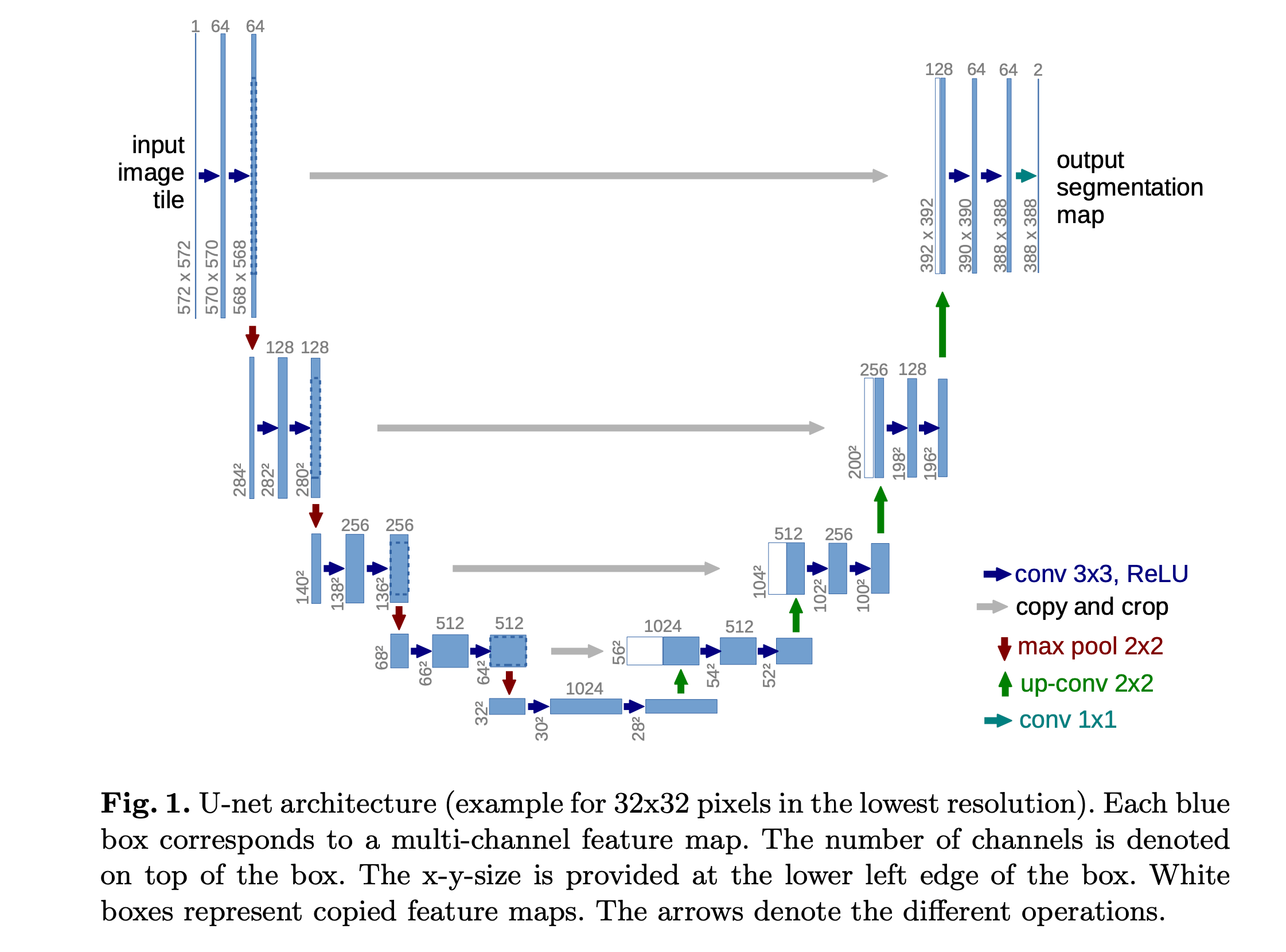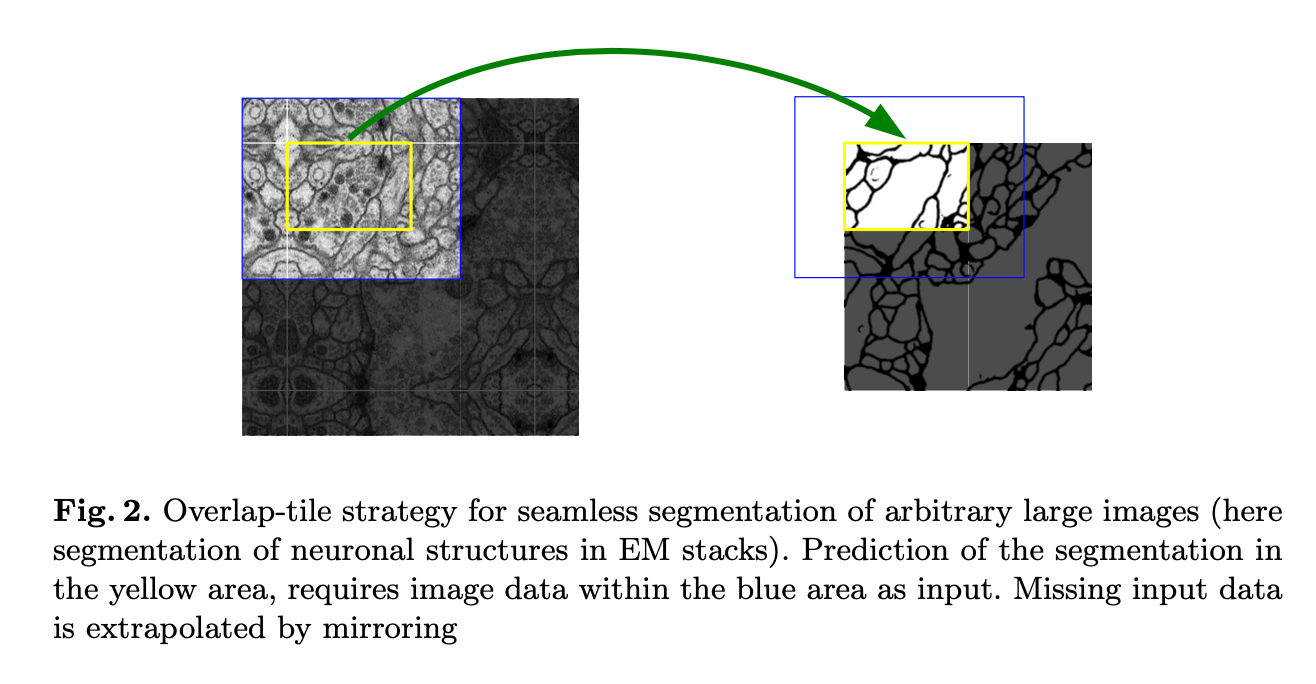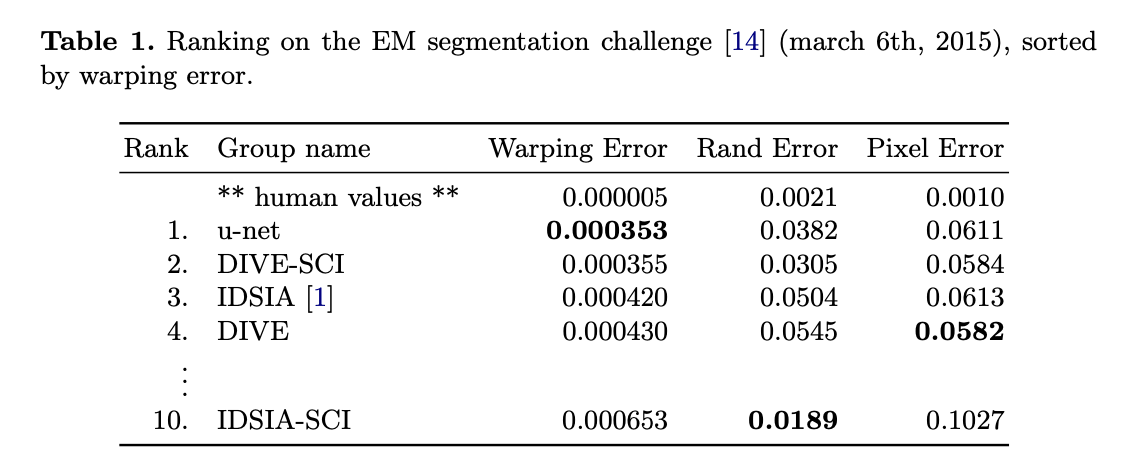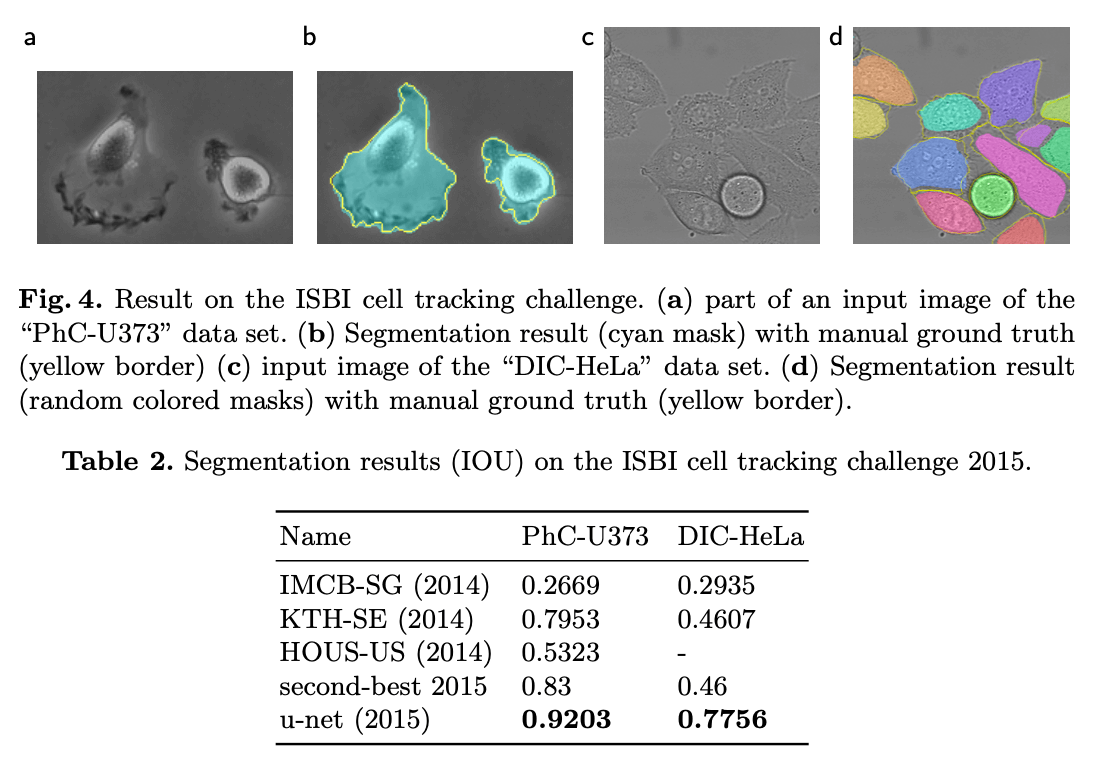# 【论文阅读】U-Net：Convolutional Networks for Biomedical Image Segmentation

## U-Net

Posted by x-jeff on March 5, 2022

# 1.IntroductionFig1中，每个蓝色块代表一个多通道的feature map，通道的数量标注在蓝色块的上方。feature map的x,y大小标注在蓝色块的左侧。白色块表示是拷贝过来的feature map。不同颜色的箭头代表不同的操作。# 3.Training

$p_k(\mathbf{x}) = exp( a_k(\mathbf{x}) ) / ( \sum^K_{k'=1} exp (a_{k'}(\mathbf{x})) )$

$a_k(\mathbf{x})$表示第$k$个feature map在某一像素坐标的激活值。feature map的通道数$K$就是类别个数，每个通道代表一个类别。经过softmax函数之后，同一像素坐标但不同通道的点只能归属于概率最大的那个类别。交叉熵损失函数定义为：

$E=\sum_{\mathbf{x} \in \Omega} w(\mathbf{x}) \log (p_{\ell (\mathbf{x})} (\mathbf{x})) \tag{1}$

$p_{\mathcal{l}(\mathbf{x})} (\mathbf{x})$为$\mathbf{x}$归属于真实类别$\ell$的概率。$w$为权重。

$w(\mathbf{x}) = w_c (\mathbf{x}) + w_0 \cdot exp \left( -\frac{(d_1(\mathbf{x}) + d_2(\mathbf{x}))^2}{2\sigma^2} \right) \tag{2}$

$w_c$是一个weight map，用于解决类别不平衡。$d_1$是到最近的细胞的边界的距离，$d_2$是到第二近的细胞的边界的距离。根据我们的经验：$w_0=10,\sigma \approx 5$。

# 4.Experiments# 5.Conclusion

u-net在生物医学分割任务中表现很好。通过弹性形变进行数据扩展使得我们仅需少量的标记数据便可以达到不错的结果。u-net的官方实现以及相关材料见：http://lmb.informatik.uni-freiburg.de/people/ronneber/u-net

# 6.原文链接